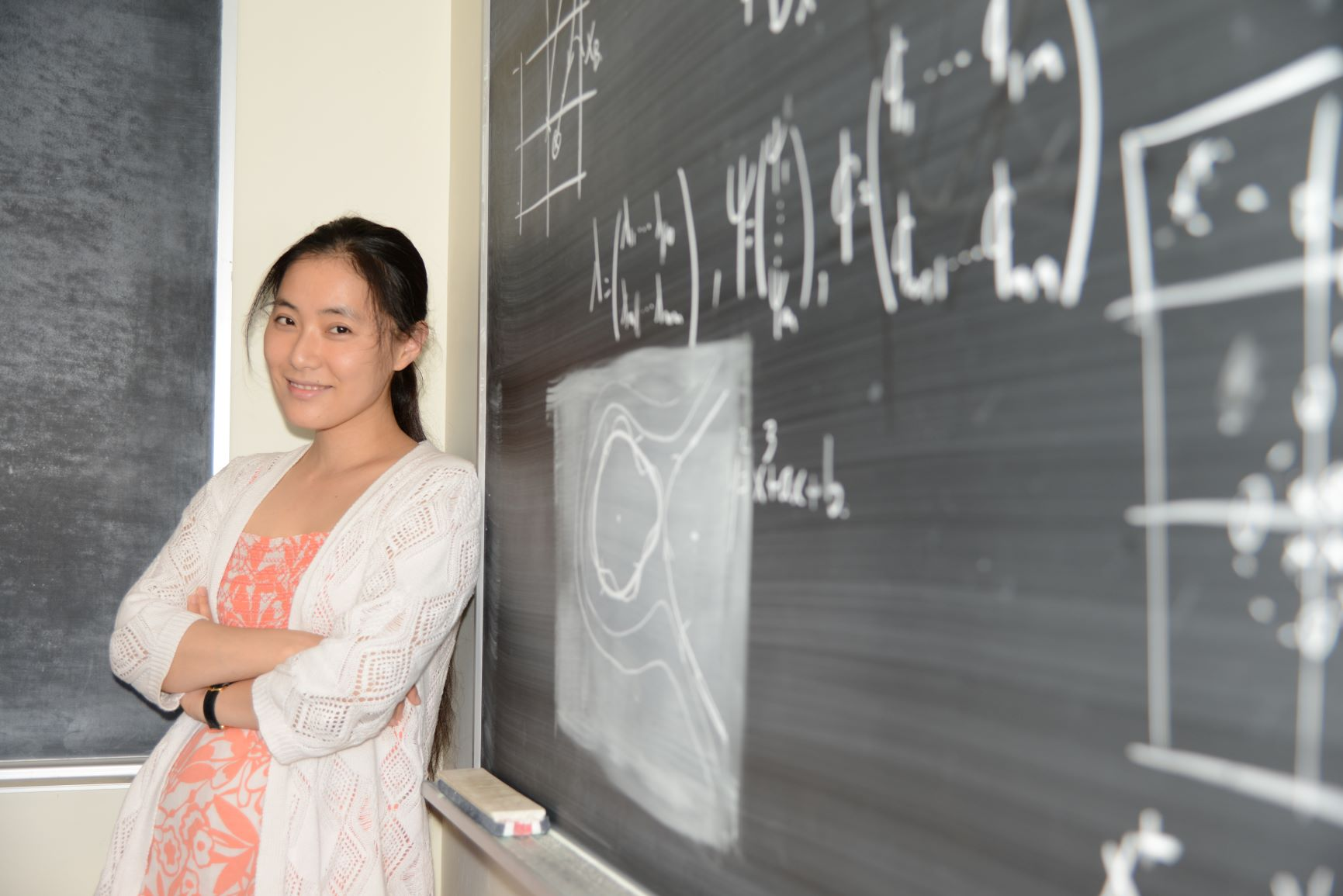• ### CNRS-Unite Mixte Internationale CNRS-UMI, 2014-present

All courses use: [MyCourses]

#### At McGill

Fall 2020
• Honours Probability (Math 356)
• Advanced Probability Theory I (Math 587)
• Winter 2021
• Advanced Probability Theory II (Math 589)

• Fall 2018
• Honours Probability (Math 356)
• Advanced Probability Theory I (Math 587)
• Fall 2017
• Honours Probability (Math 356)
• Advanced Probability Theory I (Math 587)
• Fall 2016
• Honours Probability (Math 356)
• Advanced Probability Theory I (Math 587)
• Topics in Geometry and Topology (Math 599), co-lectured with D. Jakobson.
• Fall 2015
• Calculus 1 with Precalculus (Math 139)
• Advanced Probability Theory I (Math 587)
• Honours Independent Study (Math 480) for Leila Sloman, Reinhold Willcox and Ulysse Blau.
• Winter 2015
• Honours Independent Study (Math 470) for Olivier Nadeau-Chamard.
• Fall 2014
• Calculus 1 with Precalculus (Math 139)
• Advanced Probability Theory I (Math 587)
• Fall 2013
• Calculus 1 with Precalculus (Math 139)
• Winter 2013
• Advanced Probability Theory II (Math 589)

• #### At MIT

Spring 2010
• Differential Equations (18.03)
• Fall 2009
• Multivariate Calculus (18.02)
• Spring 2009
• Differential Equations (18.03)
• Fall 2007 - Spring 2008
• TA: various undergraduate and graduate courses in probability theory and stochastic processes (18.440, 175, 177)
• #### Under Review

• X.-Y. Yin, L. Chen, J.-C Nave. A diffusion-driven Characteristic Mapping method for particle management.
[ArXiv:2008.13076]
• L. Chen and I. Weih-Wadman. Fundamental Solution to 1D Degenerate Diffusion Equation with Locally Bounded Coefficients.
[ArXiv:2008.13092]

#### Published / Accepted

• L. Chen. Steep Points of Gaussian Free Fields in Any Dimension (2020), to appear in Journal of Theoretical Probability Theory.
• L. Chen and I. Weih-Wadman. The Fundamental Solution to 1D Degenerate Diffusion Equation with One-sided Boundary, Journal of Mathematical Analysis and Applications Vol. 492, No. 1 (2020).
• L. Chen, F. Clerc and P. Panangaden. Bisimulation for Feller-Dynkin Processes, Electronic Notes in Theoretical Computer Science Vol. 347 (2019), pp 45-63.
• L. Chen and N. Shu. A Geometric Treatment of Log-Correlated Gaussian Free Fields, Contemporary Mathematics No. 739 (2019), pp 1-16.
• I. Gibbs and L. Chen. Asymptotic Properties of Random Voronoi Cells with Arbitrary Underlying Density, to appear in Advances in Applied Probability (2019).
• L. Chen. Thick points of high-dimensional Gaussian free fields, Annales. Institut Henri Poincare, Vol. 54, No. 3 (2018), pp 1492-1526.
• L. Chen and D. Jakobson. Gaussian free fields and KPZ relation in R^4, Annales Henri Poincaré, Vol. 15, No. 7 (2014), pp 1245-1283.
• L. Chen and D. Stroock. Additive functions and Gaussian measures, Prokhorov and Contemporary Probability Theory, Springer Proceedings in Mathematics and Statistics 33 (2012).
• L. Chen and D. Stroock. The fundamental solution to the Wright-Fisher equation, SIAM Journal on Mathematical Analysis, Vol. 42, No. 2 (2010), pp. 539-567.
• #### In Preparation

• L. Chen. An extension of the Liouville quantum gravity measure to polynomial-correlated Gaussian free field setting, in preparation.
• L. Chen and N. Shu, Regularization of Log-Correlated Gaussian Free Fields in Arbitrary Dimension Based on the Fourier-Bessel Expansion, in preparation.
• L. Chen. Random Measures Constructed with Polynomials of Polynomial-Correlated Gaussian Free Fields, in preparation.
• L. Chen and A. Zlotchevski, Fluctuations in the Thick Points of the Two-Dimensional Log-Correlated Gaussian Free Fields in preparation.
• L. Chen, D. Jakobson and D. Knapik, Zero Curves of Random Bivariate Polynomials, in preparation.
• #### Notes

• L. Chen. Spherical averages of Gaussian free fields, pp 1-19 -- short note (2014).
[PDF]#### Contact

Department of Mathematics and Statistics
McGill University
Burnside Hall
805 Sherbrooke West
Montreal, QC, H3A 0B9